# Square

Calculate the perimeter and the area of square with a diagonal length 30 cm.

Result

o =  84.853 cm
S =  450 cm2

#### Solution:Leave us a comment of example and its solution (i.e. if it is still somewhat unclear...):

Showing 0 comments:Be the first to comment!#### To solve this example are needed these knowledge from mathematics:

Pythagorean theorem is the base for the right triangle calculator.

## Next similar examples:

1. Diamond perimeter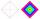Calculate the diamond circumference which area is 288 cm square and one diagonal has a size of 124 cm.
2. SquareCalculate area of the square with diagonal 64 cm.
3. HypotenuseCalculate the length of the hypotenuse of a right triangle if the length of one leg is 4 cm and its content area is 16 square centimeters.
4. The cellar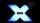Mr Novák has a cellar and a cellar window in the chalet has 0.6 meter square window. The window wishes to place an X-shaped grid in a square. He uses iron welded bars. Calculate the lengths of individual bars and what the total length of the bars he has t
5. Diagonal of squareCalculate the side of a square when its diagonal is 10 cm.
6. Playground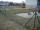Fencing square playground cost € 464; 1 meter cost € 19. What is the area of the playground?
7. Octagonal mat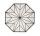Octagonal mat formed from a square plate with a side of 40 cm so that every corner cut the isosceles triangle with leg 3.6 cm. What is the content area of one mat?
8. Dog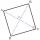Dog is tied to a chain, which is mounted in a corner of the yard. Yard has the shape of a square with a side length of 20 meters. The same long is also dogchain. Are there places in the yard where dog can't reach?
9. Square2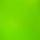Side of the square is a = 6.2 cm, how long is its diagonal?
10. Trio 2Decide whether trio of numbers is the side of a right triangle: 26,24,10.
11. Right triangle ABCCalculate the perimeter and area of a right triangle ABC, if you know the length of legs 4 cm 5.5 cm and 6.8 cm is hypotenuse.
12. RT 10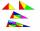Area of right triangle is 84 cm2 and one of its cathethus is a=10 cm. Calculate perimeter of the triangle ABC.
13. Rhombus 4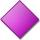Circumference of the rhombus is 44 cm, its height is 89 mm long. Calculate its content area.
14. Median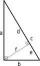In the right triangle are sides a=41 dm b=42 dm. Calculate the length of the medians tc to the hypotenuse.
15. Four ropesTV transmitter is anchored at a height of 44 meters by four ropes. Each rope is attached at a distance of 55 meters from the heel of the TV transmitter. Calculate how many meters of rope were used in the construction of the transmitter. At each attachment.
16. Chord circleThe circle to the (S, r = 8 cm) are different points A, B connected segment /AB/ = 12 cm. AB mark the middle of S'. Calculate |SS'|. Make the sketch.
17. WindbreakA tree at a height of 3 meters broke in the windbreak. Its peak fell 4.5 m from the tree. How tall was the tree?